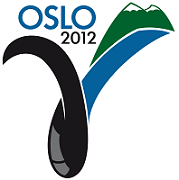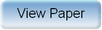Ninth International Geostatistics Congress, Oslo, Norway
June 11 – 15, 2012Session: Posters Abstract No.: P-002 Title: Approximations and bounds for binary Markov random fields Author(s): H.M. Austad, Norwegian University of Science and Technology (NO)H. Tjelmeland, Norwegian University of Science and Technology (NO) Abstract: Discrete Markov random fields (MRF) are frequently used as prior in spatial statistics, but have won less popularity in geostat applications. A severe problem with discrete MRFs is the computationally intractable normalising constant, which makes parameter estimation from a training image computationally hard. In spatial statistics applications discrete MRFs are typically used as a token prior, which is often good enough because the amount of data is sufficient for the posterior to have reasonable properties. In geostat applications the data is typically sparse, and then it is essential with a prior that really reflects the prior knowledge. In this paper we introduce an approximation scheme for binary MRFs. The basis of the approximation is the forward-backward algorithm. The exact algorithm is computationally feasible for small lattices only. It computes a series of joint marginal distributions by summing out each variable in turn. From this the normalising constant can easily be found. We represent the joint marginal distributions by interaction parameters, and make the algorithm feasible for large lattices by approximating less important interaction parameters to zero. This gives an approximation to the intractable normalising constant, and for an observed image thereby also an approximate likelihood function, Optimising this one obtains an approximation to the maximum likelihood estimator (MLE). A variant of the approximation scheme gives upper and lower limits for the normalising constant, which can be used to quantify the quality of the approximate MLE. Alternatively one may define a prior for the MRF model parameters and let the approximation define an approximate posterior distribution. Samples from this can be generated by the Metropolis--Hastings algorithm. In the paper we first give background theory for the forward-backward algorithm and our approximation scheme. Thereafter we present examples for the situations discussed above. We also discuss limitations for the proposed approximation scheme.Produced by X-CD Technologies Inc.